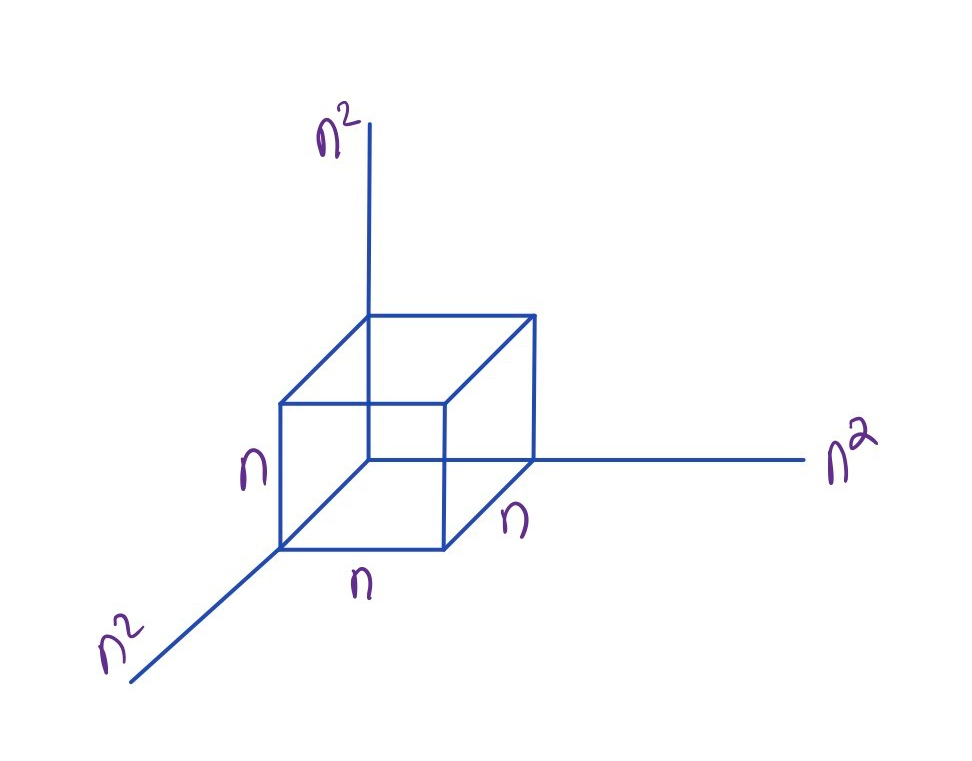# Covering sumsets and Plünnecke’s inequality

Recall Plünnecke’s inequality which states the following. We write${mA}$ for all of the${m}$–fold sums of${A}$.

Theorem (Plun): Let${A}$ be a finite subset of an abelian group. Let${K = |A+A| |A|^{-1}}$ be the doubling constant. Then$\displaystyle |mA | \leq K^{m}|A|. \ \ \ \spadesuit$

We describe one natural attempt to prove Theorem (Plun). Suppose that$\displaystyle A+A \subset A+X,$

for some small${X}$. Then$\displaystyle A+A+A \subset A+X+X.\ \ \ \ \ (1)$

and so$\displaystyle |A+A+A| \leq |A| |X|^2.$

This motivates the following definition.

Definition (Cov): Let${A}$ be a finite subset of an abelian group. We let$\displaystyle C(A) = \min \{|X| : A+A \subset A+X , \ X \subset A\}. \ \ \ \spadesuit$

Clearly${C(A) \leq |A|}$, since we may choose${|X| = |A|}$. Note that$\displaystyle C(A) \geq |A+A| |A|^{-1},\ \ \ \ \ (2)$

since if${A+A \subset A+X}$, then${|A+A| \leq |A| |X|}$. One natural question is to what extent can one reverse (2). By the argument in (1),$\displaystyle |mA| \leq C(A)^{m-1} |A|,$

and so this would have implications to Plünnecke’s inequality.

The truth is one cannot reverse (2). We give an example due to Ruzsa.

Example: We construct a set${Y}$ in${\mathbb{Z}^3}$ via$\displaystyle Y := \left( [n] \cdot e_1 \times [n] \cdot e_2 \times [n] \cdot e_3 \right) \cup [n^2] e_1 \cup [n^2] e_2 \cup [n^2] e_3.$We have the following properties of${Y}$:$\displaystyle |Y| \asymp n^3 , \ \ |Y+Y| \asymp n^4 = n |Y| , \ \ C(Y) \asymp n^2 \asymp n |Y+Y| |Y|^{-1}.$

Note that${|Y+Y|}$ comes from the sum of a line of size${n^2}$ with the box, while${C(Y)}$ comes from the sum of two perpendicular lines of size${n^2}$. The point is that one cannot efficiently cover a two dimensional object with either a one dimensional object or a three dimensional object, even in the presence of additive structure.${\spadesuit}$

We do have${C(A) \lesssim |A+A| |A|^{-1}}$ statistically in the following sense.

Proposition (Stat): Let$\displaystyle S = \{x \in A+A : r_{A+A}(x) \geq |A|^2 |A+A|^{-1}\}.$

Then$\displaystyle S \subset A+X, \ \ \ |X| \leq 3|A+A| |A|^{-1} \log |A|. \ \ \spadesuit$

This should be compared to this post of Tao, where he talks about an entropy version of Plünnecke’s inequality.

Proof: We choose${X}$ uniformly at random of size${p |A|}$. Let${d = |A|^2 |A+A|^{-1}}$. Then for${s \in S}$,$\displaystyle \mathbb{P}(s \notin A+X) = (1-p)^{r_{A+A}(s)} \leq (1-p)^{d} \leq \exp{(-pd)}.$

We choose${p = 3d^{-1} \log |A| =3 |A+A| |A|^{-2} \log |A|}$. If${p > 1}$, the Proposition follows from taking${X=A}$ and so we assume the opposite. Let${Z}$ be the random variable that denotes the number of${s \in S}$ not covered by${A+X}$. By the union bound,$\displaystyle \mathbb{E}[|Z|] \leq |A+A| \exp{(-pd)} \leq |A|^{-1}.$

Thus there is a choice of${X}$ so that${Z = 0}$ and thus${S \subset A+X}$${\spadesuit}$

On the other hand, we can bound${C(A)}$ in terms of the doubling constant if we are allowed to take${X}$ a subset of the smoother${A+A-A}$.

Proposition (Smooth): Let${A}$ be a finite subset of an abelian group. Then${A+A \subset A+X}$ where${X \subset A+A-A}$ and${|X| \ll |A+A-A||A|^{-1} \log |A|}$. Thus${A+A}$ can be covered by${\ll K^3 \log |A|}$ translates of${A}$.

The proof of Proposition (Smooth) is similar to that of Proposition (Stat), so we omit it.

This is related to the following annoying question. We let${m \cdot A}$ be the dilate of${A}$ by${m}$. We know from Plünnecke’s inequality that$\displaystyle |A+ 2 \cdot A| \leq |A+A+A| \leq K^3 |A| , \ \ K = |A+A| |A|^{-1}.\ \ \ \ \ (3)$

This inequality came up in Lemma 5.1 of the almost periodicity paper of Croot and Sisask.

Question (aplus2a): Does there exist a${\delta < 3}$ such that$\displaystyle |A+2 \cdot A| \leq K^{ \delta} |A|? \ \ \ \spadesuit$

This was asked in a paper of Bukh and appears on his website. Note that both of the inequalities in (3) can be basically tight. For the first one, consider and arithmetic progression, and for the second, the example given above.

We now give a construction that shows one cannot take${\delta}$ too small in Question (aplus2a), even for large${A}$, using the tensor power trick.

Example: Consider${A = \{0,1\}}$. Then${|A+A| = 3}$ and${|A+2 \cdot A| = 4}$. This example shows that one cannot take${\delta}$ smaller than$\displaystyle \log (2) / \log (3/2) \approx 1.71.$

To make large examples, consider${A = \{0,1\}^d}$ for some large${d}$.